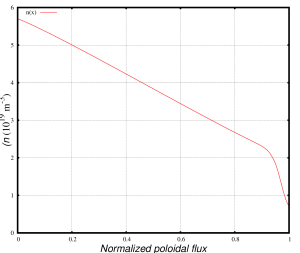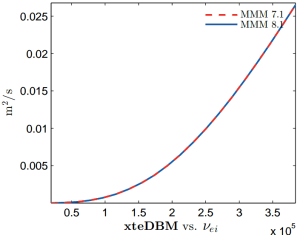## KSTAR Stability Analysis

The stability map for the KSTAR geometry is generated. The base equilibrium parameters in TOQ are the following:

rmax=47.000000
rzero=177.000000
eshape=2.00
xshape=.64000
totcur=600000.000000
baxis0=20000.000000

The base temperature and density profiles were set using parametric expressions in TOQ using the following parameters:

modelp=27
nedge13=0.7
nped13=2.0
ncore13=5.7
nfactor=1.0
tedgeEV=100.
tpedEV= 500.0
tcoreEV=2000.
widthp=.03
xphalf=.97

The corresponding density profile has the following shape:The stability map is generated by varying the nped13, tpedEV and cboot parameters the ranges [0.6:5.5], [100:1600], and [0.1:9] correspondingly. Over 150 new equilibria have been generated. The pedestal stability in these equilbria were tested using the DCON and BALOO codes. The resulting stability map is shown below:

Here$alpha$ is the normalized plasma pressure gradient defined as$alpha = -displaystyle{frac{mu_0}{2pi^2} frac{partial p}{partial psi} frac{partial V}{partial psi} sqrt{frac{V}{2pi^2 R}}},$

where$p$ is the plasma pressure,$psi$ is the poloidal flux,$V$ is the plasma volume, and$R$ is the plasma major radius.

The results are valid for a particular choice of plasma parameters such as$I_{rm pl}=0.6 MA$,$B_{rm T}=2.0 T$,$kappa=2$, and$delta=0.64$. The plasma shaping is defined according to the Bondeson’s Dee Formula. The pedestal width has been also fixed in my scan and it has been set to 0.6. The location of the H-mode pedestal top for density and temperature profiles was set to 0.94 of the normalized poloidal flux.

## Summary of plasma collisionality studies

1. The MMM8.1 model in TRANSP reproduces the experimental dependence of the energy confinement times on the plasma collisionality. However, the values of the predicted confinement times are systematically larger than the confinement times derived from experiments. Most likely reason is missing transport from modes that are not part of MMM8.1 such as microtearing modes.
2. Plasma collisionality scan using standalone MMM8.1 model revealed that most components in MMM8.1 can not explain the$tau_{rm E}(nu_star)$ dependence that is observed in predicted TRANSP simulations and in experiment. The Weiland component that computes fluxes associated with most drift-wave instabilities such as ITG modes in the plasma core shows that the electron and ion thermal diffusivities are stabilized by the plasma collisionality. This effect is expected because of known dumping effect of plasma collisionality on the drift-wave modes. There are two possible explanations of the discrepancies between predictive and standalone MMM8.1 runs.
1. In experimental plasma collisionality scans, it is very difficult to keep all dimensionless parameters unchanged. In particular, it is difficult to maintain the plasma current distribution unchanged when the plasma density changes. As result, there are notable differences in the safety factor profiles between two discharges. When the q and magnetic shear from one discharge is used in standalone runs for the other discharge, the expected$chi_e(nu_star)$ dependence is partially recovered. The differences between safety factor and magnetic shear profiles can not completely explain the differences in the$chi_e(nu_star)$ dependencies in the predictive and standalone runs, but these differences in the experimental profiles make contribution in the right direction.
2. There are modes in that DRIBM model in MMM8.1 that becomes more unstable with the resistivity. These modes are difficult to separate from other MHD modes that are described by DRIBM. However, our previous studies for DIII-D clearly show the expected$chi_e(nu_star)$ dependence:The dependence of electron thermal diffusivity on the collision frequency for DIII-D

The resistive mode in DRIBM that is found to be destabilized with the plasma collisionality is typically has large contribution in the plasma edge region near the separatrix. Note that the paleoclassical model that is distributed together with the MMM8.1 model is expected to have a similar dependence on the resistivity. However, the paleoclasical model is still being validated. Usually, it is not used in the predictive runs with the MMM8.1 model.